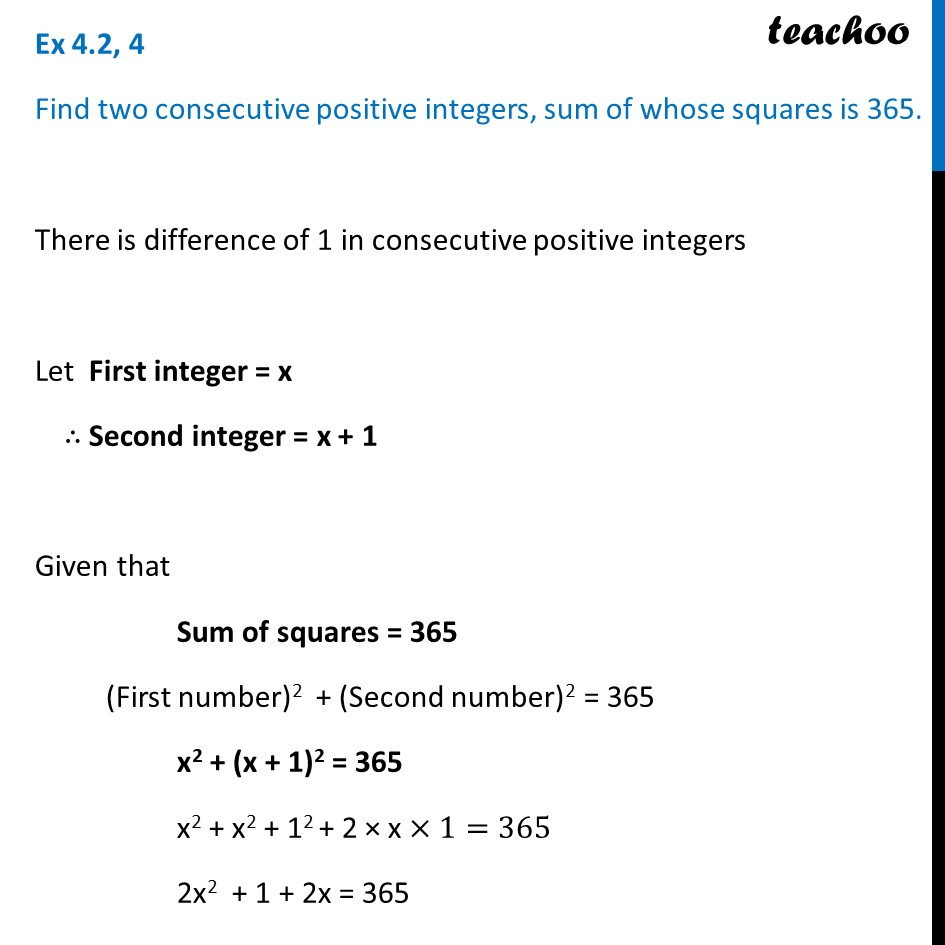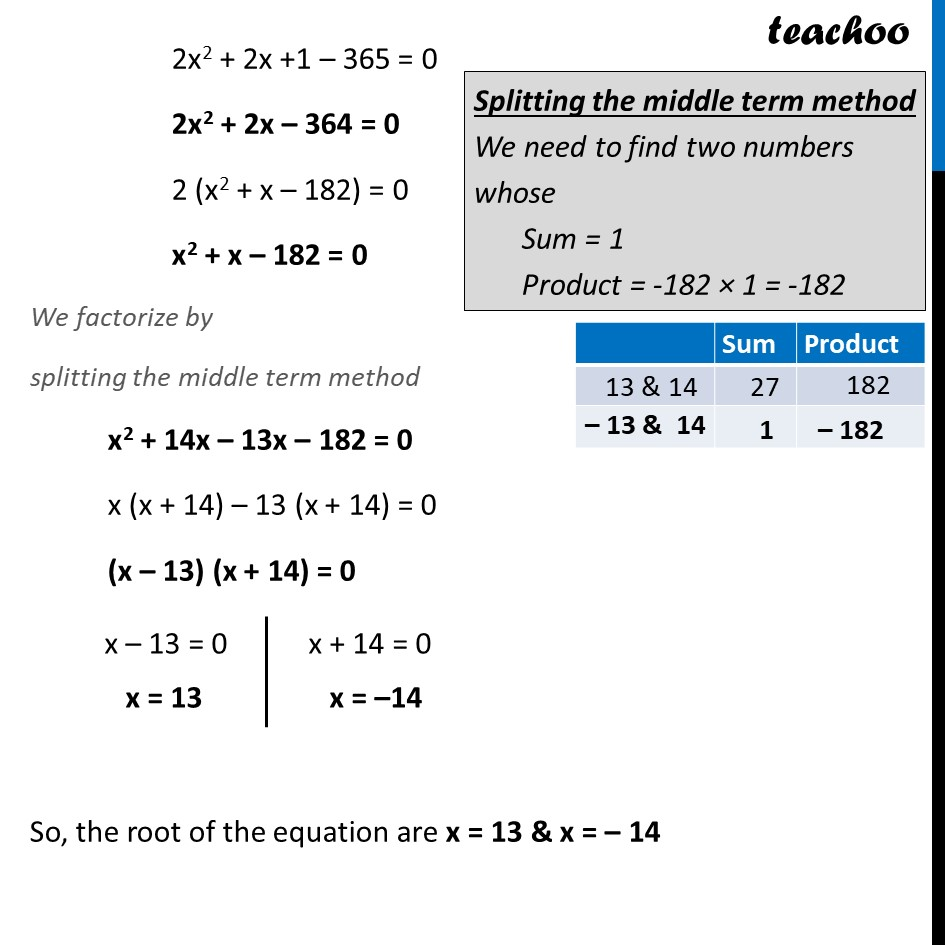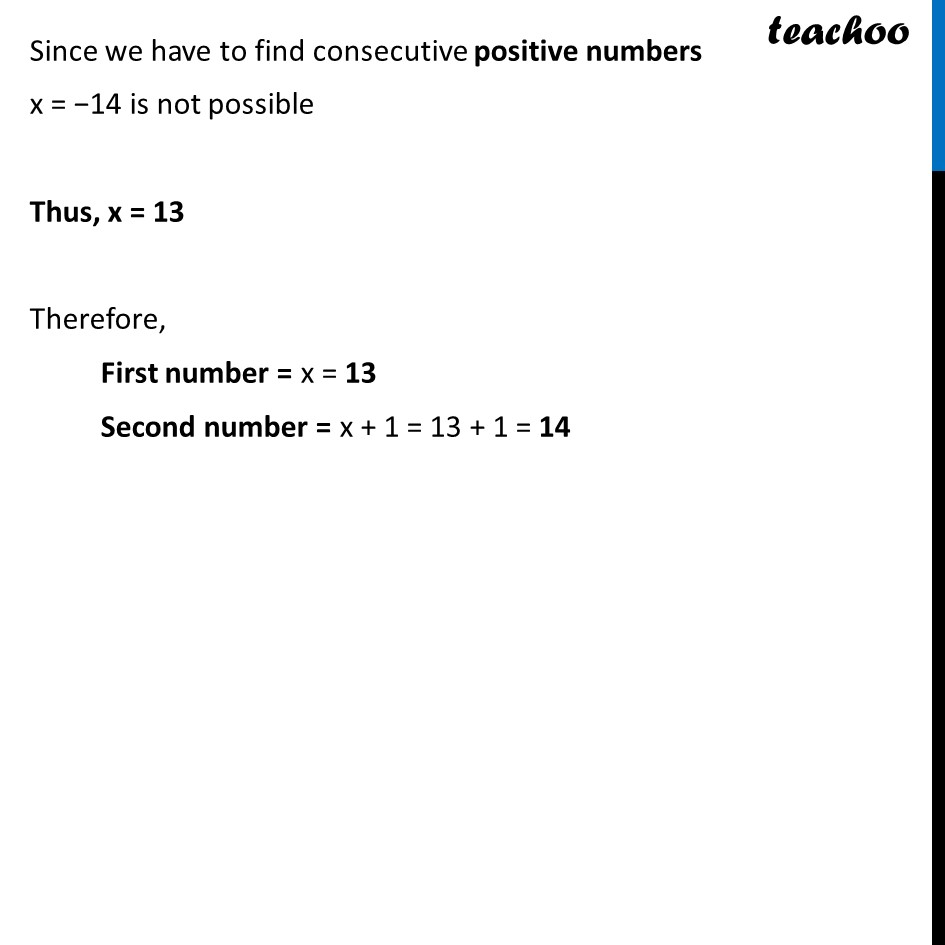Ex 4.2

Chapter 4 Class 10 Quadratic Equations
Serial order wiseLearn in your speed, with individual attention - Teachoo Maths 1-on-1 Class

### Transcript

Ex 4.2, 4 Find two consecutive positive integers, sum of whose squares is 365. There is difference of 1 in consecutive positive integers Let First integer = x ∴ Second integer = x + 1 Given that Sum of squares = 365 (First number)2 + (Second number)2 = 365 x2 + (x + 1)2 = 365 x2 + x2 + 12 + 2 × x ×1=365 2x2 + 1 + 2x = 365 x2 + x – 182 = 0/2 2x2 + 2x +1 – 365 = 0 2x2 + 2x – 364 = 0 2 (x2 + x – 182) = 0 x2 + x – 182 = 0 We factorize by splitting the middle term method x2 + 14x – 13x – 182 = 0 x (x + 14) – 13 (x + 14) = 0 (x – 13) (x + 14) = 0 So, the root of the equation are x = 13 & x = – 14 x – 13 = 0 x = 13 x + 14 = 0 x = –14 Since we have to find consecutive positive numbers x = −14 is not possible Thus, x = 13 Therefore, First number = x = 13 Second number = x + 1 = 13 + 1 = 14